Note: The other languages of the website are Google-translated. Back to English

## How to convert matrix to vector or single column in Excel?

Have you ever tried to convert a matrix of cells to a single row or column in Excel? Maybe, you can copy a row or a column and paste them one by one to a single vector, but, this will be time-consuming if there are multiple rows and columns. In this article, I will talk about some interesting methods and hope them help you.

Convert a matrix of cells to single row or column with formulas

The following formulas may help you to quickly convert the matrix to a single row or column. Please do as follows:

Convert a matrix of cells to a single column

1. First of all, you should define a range name for the matrix data. Select the range of cells and type a range name in the Name box which is next to the formula bar, and then press Enter key. In this case, I type “Matrix” as the defined name, see screenshot: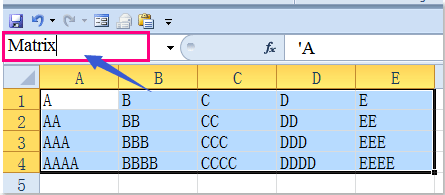2. Then enter the following formulas:

(1.) Convert the matrix to single column based on row, it means the values are taken from each row, moving across then down: =OFFSET(Matrix,TRUNC((ROW()-ROW(\$G\$1))/COLUMNS(Matrix)),MOD(ROW()-ROW(\$G\$1),COLUMNS(Matrix)),1,1)(Matrix is the range name you defined in step 1, and G1 is the cell you enter this formula). Then drag the fill handle to the cells till 0 is displayed, see screenshot: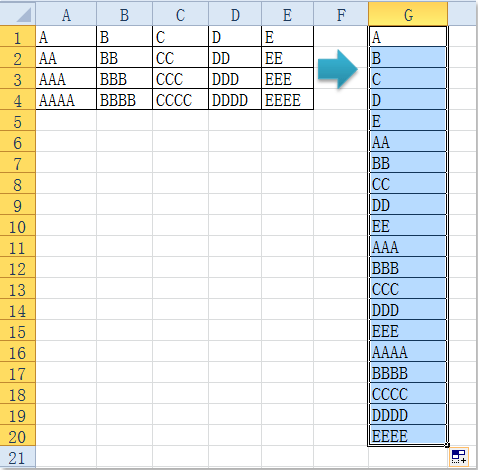(2.) Convert the matrix to single column based on column, it means the values are taken from columns, moving down one column and then across to the right column: =OFFSET(Matrix,MOD(ROW()-ROW(\$G\$1),ROWS(Matrix)),TRUNC((ROW()-ROW(\$G\$1))/ROWS(Matrix)),1,1) (Matrix is the range name you defined in step 1, and G1 is the cell you enter this formula). Then drag the fill handle to the cells till 0 is displayed, see screenshot: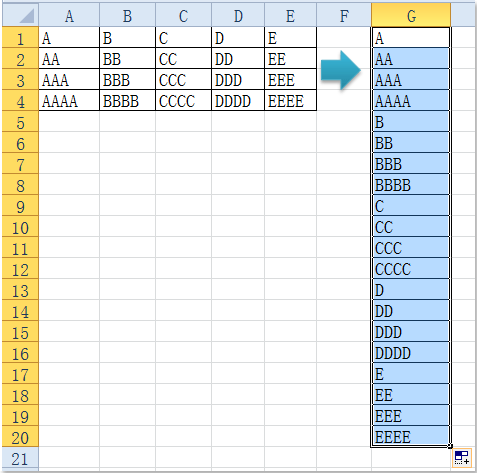Convert a matrix of cells to a single row

To convert a matrix of cells to a single row, you can use the following formulas:

(1.) Convert the matrix to single row based on row, it means the values are taken from each row from left to right on one row and then moving down to the next row: =OFFSET(Matrix,TRUNC((COLUMN()-COLUMN(\$A\$7))/COLUMNS(Matrix)),MOD((COLUMN()-COLUMN(\$A\$7)),COLUMNS(Matrix)),1,1) (Matrix is the range name you created for your data range, and A7 is the cell you enter this formula). Then drag the fill handle right to the cells till 0 is displayed, see screenshot: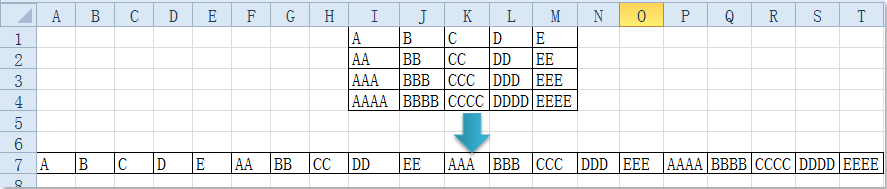(2.) Convert the matrix to single row based on column, it means the values are taken from each column, moving down one column and then across to the right column: =OFFSET(Matrix,MOD((COLUMN()-COLUMN(\$A\$7)),ROWS(Matrix)),TRUNC((COLUMN()-COLUMN(\$A\$7))/(ROWS(Matrix))),1,1) (Matrix is the range name you created for your data range, and A7 is the cell you enter this formula). Then drag the fill handle right to the cells till 0 is displayed, see screenshot: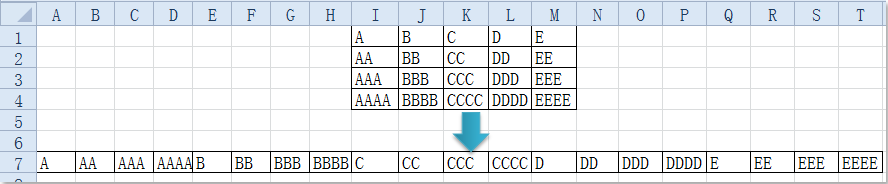If the above formulas are too long to remember, here, I can recommend an easy and powerful tool – Kutools for Excel, with its Transform Range utility, you can quickly convert multiple columns and rows to a single column or row as you need.

 : with more than 300 handy Excel add-ins, free to try with no limitation in 30 days.

After installing Kutools for Excel, please do as following steps:

1. Select the matrix of cells that you want to convert.

2. Then click Kutools > Range > Transform Range, see screenshot: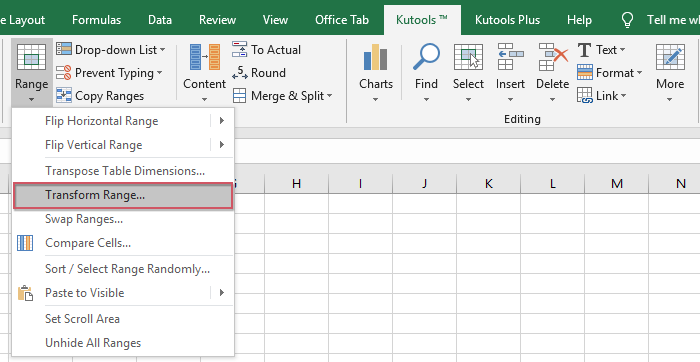3. In the Transform Range dialog, select Range to single column if you want to convert the matrix to a single column, or choose Range to single row if you want to convert matrix to a single row, see screenshot: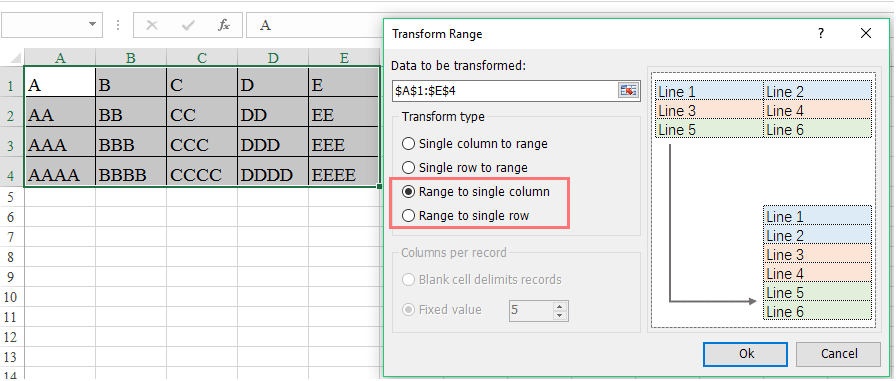4. Then click OK button, and in the popped out Transform Range box, select a cell where you want to put the result, see screenshot: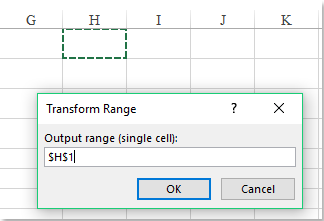5. And then click OK button, your select matrix of cells have been converted a single row or column.

Kutools for Excel: with more than 300 handy Excel add-ins, free to try with no limitation in 30 days.

### The Best Office Productivity Tools

#### Kutools for Excel Solves Most of Your Problems, and Increases Your Productivity by 80%

• Reuse: Quickly insert complex formulas, charts and anything that you have used before; Encrypt Cells with password; Create Mailing List and send emails...
• Super Formula Bar (easily edit multiple lines of text and formula); Reading Layout (easily read and edit large numbers of cells); Paste to Filtered Range...
• Merge Cells/Rows/Columns without losing Data; Split Cells Content; Combine Duplicate Rows/Columns... Prevent Duplicate Cells; Compare Ranges...
• Select Duplicate or Unique Rows; Select Blank Rows (all cells are empty); Super Find and Fuzzy Find in Many Workbooks; Random Select...
• Exact Copy Multiple Cells without changing formula reference; Auto Create References to Multiple Sheets; Insert Bullets, Check Boxes and more...
• Extract Text, Add Text, Remove by Position, Remove Space; Create and Print Paging Subtotals; Convert Between Cells Content and Comments...
• Super Filter (save and apply filter schemes to other sheets); Advanced Sort by month/week/day, frequency and more; Special Filter by bold, italic...
• Combine Workbooks and WorkSheets; Merge Tables based on key columns; Split Data into Multiple Sheets; Batch Convert xls, xlsx and PDF...
• More than 300 powerful features. Supports Office / Excel 2007-2021 and 365. Supports all languages. Easy deploying in your enterprise or organization. Full features 30-day free trial. 60-day money back guarantee.#### Office Tab Brings Tabbed interface to Office, and Make Your Work Much Easier

• Enable tabbed editing and reading in Word, Excel, PowerPoint, Publisher, Access, Visio and Project.
• Open and create multiple documents in new tabs of the same window, rather than in new windows.
• Increases your productivity by 50%, and reduces hundreds of mouse clicks for you every day!No ratings yet. Be the first to rate!
This comment was minimized by the moderator on the site
This is so useful! The formula to convert a matrix to single column based on column was exactly what I needed. Thank you!
This comment was minimized by the moderator on the site
I'd like to accomplish this same task but with a range that is not so neatly defined. I've got wholes in the data and my matrix is spaced out over my spreadsheet rather than in a neatly define A1:D4 style block. Any ideas on how I can accomplish this?
This comment was minimized by the moderator on the site
I had the same issue, put a filter on that column, and anything that has 0, filter out.
This comment was minimized by the moderator on the site
Hi, Kevin:

The following VBA code can help you to combine the cells from a range:

Sub a()
Dim xSRg As Range
Dim xDRg As Range
Dim I As Long
Dim J As Long
Dim K As Long
Dim xArr
On Error Resume Next
Set xSRg = Application.InputBox("Please select the data range:", "KuTools for Excel", Selection.Address, , , , , 8)
If xSRg Is Nothing Then Exit Sub
Set xDRg = Application.InputBox("Select an output cell:)", "KuTools for Excel", , , , , , 8)
If xDRg Is Nothing Then Exit Sub
xArr = xSRg
K = 0
For I = 1 To UBound(xArr, 1)
For J = 1 To UBound(xArr, 2)
If xArr(I, J) <> "" Then
xDRg.Offset(K, 0).Value = xArr(I, J)
K = K + 1
End If
Next
Next
End Sub

This comment was minimized by the moderator on the site
Thank you!!!!
This comment was minimized by the moderator on the site
Fantastic explanation - thank you. This helped me save hours of time
This comment was minimized by the moderator on the site
Thanks much! Stacking a 2D matrix of data as a 1D column -> That was cool! Solved my problem precisely. Though I had to tweak the formula to get it right (I think the row and col are interchanged)... But the inspiration was from this article. Simply superb! Thanks!
There are no comments posted here yet